﻿ Effects of Atypical Observations on the Estimation of Seemingly Unrelated Regression ModelPublications are Open
Access in this journal
Article Versions
Export Article
• Normal Style
• MLA Style
• APA Style
• Chicago Style
Research Article
Open Access Peer-reviewed

### Effects of Atypical Observations on the Estimation of Seemingly Unrelated Regression Model

A. A. Adepoju , A. O. Akinwumi
Journal of Mathematical Sciences and Applications. 2017, 5(2), 30-35. DOI: 10.12691/jmsa-5-2-1
Published online: October 14, 2017

### Abstract

The Seemingly Unrelated Regression Equation model is a generalization of a linear regression model that consists of several regression equations in order to achieve efficient estimates. Unfortunately, the assumptions underlying most SUR estimators give little/no consideration to outlying observations which may be present in the data. These atypical observations may have some apparent distorting effects on the estimates produced by these estimators. This study thus examined the effect of outliers on the performances of SUR and OLS estimators using Monte Carlo simulation method. The Cholesky method was used to partition the variance-covariance matrix by decomposing it into the upper and lower non-singular triangular matrices. Varying degree of outliers; 0%, 5%, and 10% were each introduced into five sample sizes; 20, 40, 60, 100 and 500 respectively. The performances of the estimators were evaluated using Absolute Bias (ABIAS) and Mean Square Error (MSE). The results showed that at 0% outliers (when outliers were absent), the ABIAS and MSE of the SUR and OLS estimators showed similar results. At 5% and 10% outliers, the magnitude in ABIAS and MSE for both estimators increased but the SUR estimator showed better performance than the OLS estimator. As the sample size increases, ABIAS and MSE of the estimators decreased consistently for the various degrees of outliers considered with SUR consistently better than OLS.

### 1. Introduction

“Seemingly unrelated regression equations” is an expression first used by Zellner 1. It indicates a set of equations for modelling the dependence of m variables (m ≥ 1) on one or more regressors in which the error terms in the different equations are allowed to be correlated and, thus, the equations should be jointly considered 2. Thus, SUR models are often applied when there may be several equations, which appear to be unrelated; however, they may be related by the fact that: (1) some coefficients are the same or assumed to be zero; (2) the disturbances are correlated across equations; and/or (3) a subset of right hand side variables are the same.

Since their introduction, SUR models have taken an important place in econometrics and in statistics. See for example 3. Srivastava & Giles 4 give a detailed treatment of estimation and inference in SUR models. However, since the procedure proposed originally by Zellner 1 is essentially a least squares estimator in a multiple equations model with a particular covariance matrix, it is expected that the estimator is vulnerable to outliers.

Seemingly unrelated regression models have been studied through many approaches. In Zellner 1, 5 feasible generalized least squares estimators are introduced and their properties are analysed. The maximum likelihood estimator from a Gaussian distribution for the error terms is investigated, for example, in Kmenta and Gilbert 6; Oberhofer and Kmenta 7; Magnus 8; Park 9. Further developments have been obtained by using bootstrap methods 10, 11.

The Seemingly Unrelated Regression (SUR) estimator deals with a system of multivariate equations when error variables are contemporaneously correlated. There are two main motivations for use of SUR. The first one is to gain efficiency in estimation by combining information on different equations. The second motivation is to impose and/or test restrictions that involve parameters in different equations. Zellner 1 provided the seminal work in this area, and a thorough treatment is available in the book by Srivastava and Giles 4. When contemporaneous correlations between the disturbances are high and the explanatory variables in different equations considered are uncorrelated then efficiency would be attained 1, 12, 13, 14, 15.

The OLS solutions of the seemingly unrelated regression model ignores any correlation among the errors across equations; however, because the dependent variables are correlated and the design matrices may contain some of the same variables, there may be contemporaneous correlation among the errors across the equations. The Seemingly Unrelated Regression (SUR) estimator is efficient as it takes into account the covariance structure of the errors 16, 17.

Zeller 1 showed that univariate analysis may result in inefficient estimates of the covariate effects. SUR is a special case of the multivariate regression model that is used to capture the effect of different covariates allowed in the regression equations. It is a joint modeling where systems of equations are considered being related through error. Zeller concluded that SUR models allow for simultaneous estimation of multiple models with different covariables while accounting for the correlated errors.

Binkley 16 examined the effect of variable correlation on an individual coefficient estimated by SUR in a two-equation model. It was seen that the effect of correlation among variables across the equations greatly depends on the multicollinearity already existing within an equation. It was noted that the major factor determining the efficiency gain of SUR for the coefficient on an individual variable is not the correlation between that variable and those in the other equation. Rather, it is the correlation between the latter and the residuals obtained by regressing the variable in question on the remaining variable in its own equation.

An outlier is defined as an observation that appears to be inconsistent with other observations in a data set. They can occur by chance in a distribution but are mostly indicative of measurement error which one can decide to discard or use statistics that are robust. They can also come from incorrect specifications that are based on the wrong distributional assumptions at the time the specifications are generated. They provide useful information about a process 18. In practice, data collected in a broad range of applications frequently contain one or more atypical observations called outlier. A single outlier can have a large distorting influence on a classical statistical method that is optimal under the assumption of normality or linearity. Many estimation procedures proposed by researchers to handle SUR equation models are based on the assumptions that give little consideration to atypical data, however, these atypical observations may have some apparent distorting effects on the estimates produced by these estimators, thus the need to investigate the distorting effects of outliers in seemingly unrelated regression equations estimation methods.

The presence of outlier in a data set can lead to inflated error rates and substantial distortions of parameter and statistic estimates when using parametric or nonparametric test 19. The effects of outliers will pervade through all the equations and the estimated parameters in them. These effects are so intricately pervasive that it is very difficult to assess the influence of outliers on the estimated parameters 20. 21 confirmed empirically that researchers rarely report checking for outliers of any sort, by reporting that authors reported testing assumptions of the statistical procedure(s) used in their studies, including checking for the presence of outliers, only 8% of the time 22.

The assumptions underlying most SUR estimators give little consideration to influential observations which may be present in the data; however, these atypical observations may have some obvious distorting effects on the estimates produced by these estimators 23. Moreover, the effects of outliers may permeate through the system of equations, the primary aim of SUR which is to achieve efficiency in estimation is therefore questionable. The aim of this study is therefore to investigate the effect of outliers on the performances of equation-by-equation application of least squares (OLS) and SUR estimator (FGLS) and to examine the asymptotic properties of the estimators in the presence of outliers.

The rest of the paper is organised as follows: Section 2 illustrates the theory behind the methodology followed by the design of the simulation experiment in section 3. Analysis and Discussion of results as presented in sections 4 and 5. In Section 6 some concluding remarks are provided.

### 2. Materials and Methods

The system of m seemingly unrelated regression equations can be stacked in two equivalent compact matrix forms. It can be expressed as a multiple linear regression model: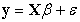(1)

Where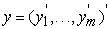is the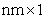response vector,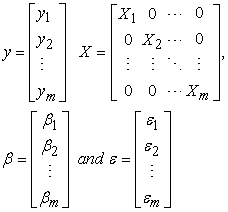(2)

X is the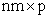structured design matrix, with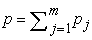,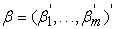is the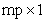parameter vector. It is namely assumed that the error vectors are contemporaneously but not serially correlated herein. This means that for given observations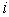and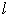, across the regression equations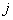and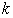, holds that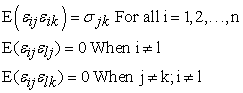(3)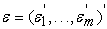is the error vector with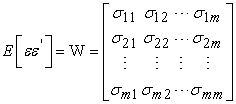(4)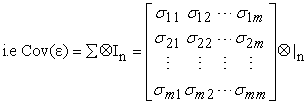(5)

The above equation (1) can also be estimated separately using the OLS estimator but this would ignore the covariance structure of the errors. A more efficient estimator is obtained as the GLS estimator with weight matrix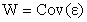and we have,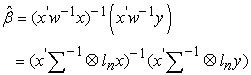(6)

From above,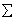is unknown, therefore a Feasible GLS (FGLS) estimator also called the SUR estimator is preferred that replaces the unknown W with a consistent estimate. The FGLS estimator is an iterative two-step procedure that uses estimates for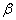to estimate, which is then used to improve the regression estimates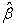.

Each equation is estimated by OLS, giving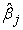.

The residuals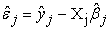from the m equations are used to estimate the error covariance matrix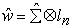with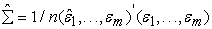(7)

New estimates ofare obtained, known as the feasible generalized least squares (FGLS) as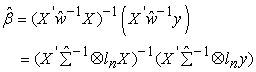(8)

The estimated covariance matrix ofis given by,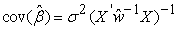(9)

### 3. Design of the Simulation Experiment

A three-equation of seemingly unrelated regression model with correlated errors is given as;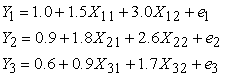(10)

Where n = 1, 2, … , N (number of observations)

In each equation, the explanatory variables were contaminated with various degrees of outliers leading to the contamination of the whole equation. Contamination was done at 0%, 5% and 10% respectively. The Monte Carlo Simulation was used to simulate the explanatory variables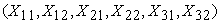from a normal distribution for various sample sizes N=20, 40, 60, 100, and 500.

The following data were generated following the steps below;

The vectors of the X’s independent regressors were generated by drawing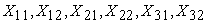from a normal distribution and contaminated at varying degrees of outliers (0%, 5% and 10%).

The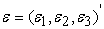, are series of random normal deviates which were standardized and appropriately transformed to ensure that the disturbance terms are contemporaneously correlated and have specific variance-covariance matrices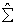estimated in the model

From definition,is a definite matrix, therefore there exists a non- singular triangular matrix P such that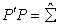The estimated variance-covariance matrixwas obtained and later decomposed into upper and lower non-singular triangular matrices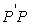such that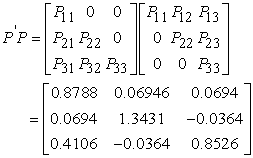(11)

Solving for the unknowns in equation (11), we have;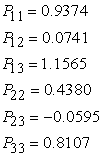(12)

Thus, the Cholesky (variance-covariance) decomposition for N=20 is computed as;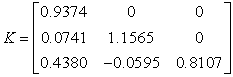(13)

To establish a strong comtemporaneous relationship between the three sets of equations when N=20) through their error terms, we have,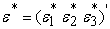in place of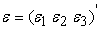whose elements are determined by the product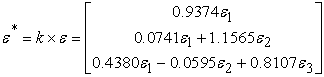(14)

Since certain values have been assigned to the structural parameters, we pre-determined the coefficients as in Monte Carlo experiment. The experiment was carried out for each of the sample sizes. Codes were written using the R- software following the above procedures.

The known parameters were then estimated as unknowns using SUR and OLS estimation methods. The performances of both estimators were compared.

### 4. Analysis of Simulated Results

The summary of the results of the simulation described above when the SUR model is contaminated with varying degrees of outliers, 0%, 5% and 10% at various sample sizes N=20, 40, 60, 100, and 500 are presented in the Tables below. At mentioned earlier, the performances of OLS and SUR estimators were adjudged using ABIAS and MSE.

From Table 1, the OLS estimator showed consistent asymptotic behaviour with the values of MSE decreasing consistently as the sample size increases from N = 20 to 500 for all the equations at the various percentage of contamination. The values of ABIAS on the other hand, also decrease consistently for the levels of contamination considered as the sample size increases except for Equation 1 at 0% contamination at N = 20 and N = 40 respectively. However, for Equation 2 the same values are obtained for ABIAS at N = 20 and N = 40 for all percentage of contamination. It is worth noting that both ABIAS and MSE estimates decrease as the percentage contamination increases for all the sample sizes.

From Table 2, the ABIAS estimates obtained at N = 20 for Equation 1 is the same for 0% and 5% contamination levels. For Equation 2, the ABIAS estimates at N = 20 and N = 40 are exactly the same for 0% and 5% levels of contamination. The SUR estimator exhibits an asymptotic behaviour with the values of both ABIAS and MSE consistently decreasing at all the levels of contamination under consideration as the sample size increases. The SUR estimator is not greatly affected by the presence of outliers at the large sample sizes (i.e. N = 100 and 500) even as the percentage contamination is increased. The values of ABIAS and MSE increase as the contamination level increases for all the sample sizes and Equations but at small magnitudes for large sample sizes.

Table 1 and Table 2 are combined to produce Table 3 to facilitate comparison across estimators, sample sizes and percentage outliers.

As the percentage of outlier increases, the ABIAS and MSE of both estimators increase drastically for all the sample sizes. The effect of the presence of outliers is not pronounced at large sample sizes, specifically at N = 100 and 500, as values of both ABIAS and MSE in most cases decrease as sample size increases for OLS while the values of ABIAS and MSE consistently decrease for SUR revealing asymptotic property of the SUR estimator. The results also point out that the ABIAS and MSE of the OLS estimates are larger than the SUR especially at N = 60, 100 and 500.

### 5. Discussion of Results

From the Tables, in the absence of outliers, the mean square error and the absolute bias of the SUR and OLS estimators showed similar performances in all the models. In the presence of 5% outliers, there was an increase in the mean square error and the absolute bias of the estimators while at 10% there was a further increase in the absolute bias and the mean square error of the estimators considered but the SUR estimator showed better performance than the OLS estimator in all the equations.

It is also observed that the mean square error of the SUR and the OLS estimators consistently increased in the presence of outliers while they maintained a normal balance in the absence of outliers. However, the larger the sample size, the lower the effect of the outliers on the estimators. The SUR estimator however performed consistently better than the OLS estimator.

### 6. Conclusion

The main focus of this paper is to determine a better method of estimating the parameters of seemingly unrelated regression in the presence of outliers. The empirical study reveals that the OLS estimates are easily affected by the outliers especially at small sample sizes hence it is not reliable. On the other hand, the SUR estimates emerge to be conspicuously more efficient and more reliable as it is less affected by the outliers.

This study found out that the mean square error and the absolute bias estimates of the SUR estimator (FGLS) were generally smaller than the mean square error and absolute bias of the OLS estimator in the presence of varying degrees of outliers, however in the absence of outliers, the estimators showed similar performances.

### References

  Zellner A. (1962): An Efficient Method of Estimating Seemingly Unrelated Regression Equations and Tests of Aggregation Bias, Journal of the American Statistical Association, 57 (298): 348-368; 500-509. In article View Article  Galimberti Giuliano, Scardovi Elena, Soffritti Gabriele (2014): Using mixtures in seemingly unrelated linear regression models with non-normal errors, Department of Statistical Sciences, University of Bologna. In article View Article  Judge, G. G., Hill, R. C., Grittiths, W. E., Liitkepohl, H. and. Lee, T.. C. (1985): The Theory and Practice of Econometrics, 2nd Edition. Wiley, New York. In article View Article  Srivastava, V. K. and D. E. A. Giles (1987): Seemingly Unrelated Regression Equations Models, New York: Marcel Dekker Inc. In article View Article  Zellner, A. (1963): Estimators for seemingly unrelated regression equations: some exact finite sample results. J. Am. Stat. Assoc. 58, 977-992. In article View Article  Kmenta, J., Gilbert, R. (1968): Small sample properties of alternative estimators of seemingly unrelated regressions. J. Am. Stat. Assoc. 63, 1180-1200. In article View Article  Oberhofer, W., Kmenta, J. (1974): A general procedure for obtaining maximum likelihood estimates in generalized regression models. Econometrica 42, 579-590. In article View Article  Magnus, J. R. (1978): Maximum likelihood estimation of the GLS model with unknown parameters in the disturbance covariance matrix. J. Econom. 7, 281-312. In article View Article  Park, T. (1993): Equivalence of maximum likelihood estimation and iterative two-stage estimation for seemingly unrelated regression models. Commun. Stat. Theory 22, 2285-2296. In article View Article  Rocke, D. (1989): Bootstrap Bartlett adjustment in seemingly unrelated regression. J. Am. Stat. Assoc. 84, 598-601. In article View Article  Rilstone, P., Veall, M. (1996): Using bootstrapped confidence intervals for improved inferences with seemingly unrelated regression equations. Econom. Theory 12, 569-580. In article View Article  Binkley, J.K. and Nelson, C.H. (1988): a note on the efficiency of seemingly unrelated regression. The American Statistician 42(2): 137-139. In article View Article  Conniffe, D. (1982): A note on seemingly unrelated regressions. Econometrica 50(1): 229-233. In article View Article  Moon, Hyungsik Roger and Perron, Benoit (2006): seemingly unrelated regression Models. In article  Viraswami k. (1998): Some efficiency results on Seemingly Unrelated Regression Equations. In article View Article  Binkley J. K, (1982): Journal of the American Statistical Association Vol 77:890-895. In article View Article  Bartels, R. and Fie big, D.G. (1991): A simple characterization of seemingly unrelated regressions model in which OLS is BLUE. The American Statistician 45(2): 137-140 5. In article View Article  Rousseeuw P.J. and Leroy A.M. (1987): Robust Regression and Outlier Detection. In article View Article  Zimmerman, D. W. (1998): Invalidation of parametric and nonparametric statistical tests by concurrent violation of two assumptions. Journal of Experimental Education, 67 (1), 55-68. In article View Article  Mishra, S. K. (2008): Robust Two-Stage Least Squares: Some Monte Carlo Experiments. MPRA Paper No. 9737M. (Online at http://mpra.ub.uni-muenchen.de/9737/) In article View Article  Osborne, J. W, Christian, W. R. I, & Gunter, J. S. (2001): Educational psychology from a statistician’s perspective: A review of the quantitative quality of our field. Paper presented at the Annual Meeting of the American Educational Research Association, Seattle, WA. In article View Article  Adepoju, A. A. and Olaomi, J. O. (2012): Evaluation of Small Sample Estimators of Outliers Infested Simultaneous Equation Model: A Monte Carlo Approach. Journal of Applied Economic Sciences Vol. 7. No. 1: 8-16. In article View Article  Oseni, B. M. and Adepoju, A. A. (2011): Assessment of Simultaneous Equation Techniques under the Influence of Outliers. Journal of The Nigerian Statistical Association Vol. 23: 1-9. In articleThis work is licensed under a Creative Commons Attribution 4.0 International License. To view a copy of this license, visit http://creativecommons.org/licenses/by/4.0/